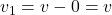## Let two objects of equal mass m collide. Object 1 has initial velocity v, directed to the right, and object 2 is initially stationary.

Question

Let two objects of equal mass m collide. Object 1 has initial velocity v, directed to the right, and object 2 is initially stationary.

A. If the collision is perfectly elastic, what are the final velocities v1 and v2 of objects 1 and 2?

Give the velocity v1 of object 1 followed by the velocity v2 of object 2, separated by a comma. Express each velocity in terms of v.

in progress 0
6 months 2021-08-19T19:08:28+00:00 1 Answers 0 views 0

## Answers ( )

(v, 0) or (0, v)

Explanation:

The law of conservation of linear momentum states total initial momentum equal total final momentum.

Total initial momentum =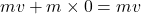Total final momentum =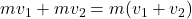Equating both,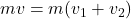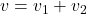For an elastic collision, kinetic energy is conserved i.e. total initial kinetic energy = total final kinetic energy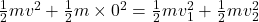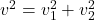From the first equation,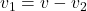.

Substituting this in the second equation,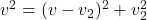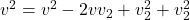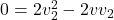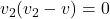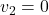or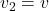From,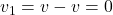OR